# FFT Analyzer

FFT analyzer manual can be found here.

When you press the Setup button on FFT analyser, the following setup window will open: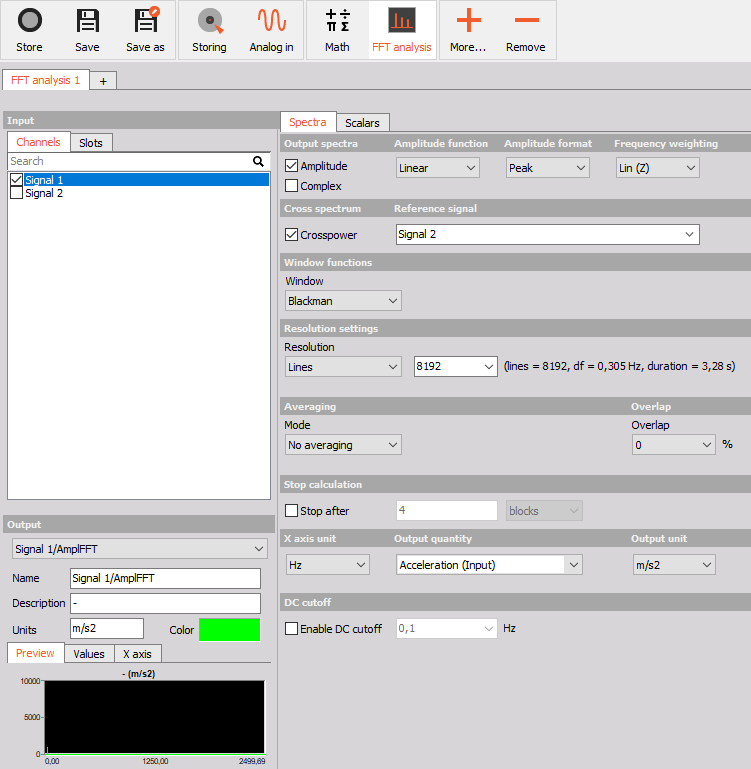## Output spectra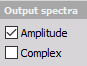• Complex - outputs are phase, imaginary and real part of the signal.
• Amplitude - output is the amplitude of the signal.

### Amplitude function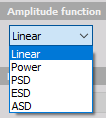In Amplitude function we can choose from:

• Linear - is the pure signal amplitude [V].
• Power - calculated as RMS value squared [V*V] (also known as AutoPower)
• PSD - calculated as RMS squared, divided by the line resolution and sqrt(2), used for checking the noise [V*V/Hz].
• ESD - energy distribution for each unit frequency.
• ASD - acceleration spectral density.

Amplitude function has no effect on the complex spectrum. Only the amplitude is scaled to the complex spectrum according to the format amplitude setting (RMS, peak, peak-peak).

Amplitude function only affects the amplitude spectrum. Linear means that the amplitude of the spectrum is expressed in the same units as the input signal.

It is calculated as the absolute value of the complex spectrum, which, like the complex spectrum, is additionally scaled according to the format amplitude setting. Unlike the complex spectrum, the amplitude spectrum can also be averaged over the entire measurement or at intervals with ordinary or energy averages.

Amplitude spectrum has no phase information but only the complex does.

### Amplitude format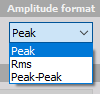The Amplitude format display section defines display in Y-amplitude axis.

In Amplitude type we can choose from:

• Peak - is the pure signal amplitude [V].
• RMS - is the RMS amplitude, calculated as Amplitude/sqrt(2) [V].
• Peak-peak - is the signals peak-peak amplitude [V].

### Frequency weighting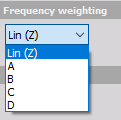As a standard, FFT analyser uses Linear Weighting. For sound analysis, special FFT weighting can be set. As opposed to the sound module in math, where the weightings will be calculated in the time domain, this will calculate the sound weighting in the frequency domain. Weighting can be of the following type:

• Linear weighting - is linear at all frequencies and it has the same effect on all measured values.
• A weighting - is applied to instrument-measured sound levels in an effort to account for the relative loudness perceived by the human ear, as the ear is less sensitive to low audio frequencies.
• B weighting - is used for intermediate levels and is similar to A, except for the fact that low-frequency attenuation is a lot less extreme though still significant (-10 dB at 60 Hz). This is the best weighting to use for music listening purposes.
• C weighting - is similar to A and B as far as the high frequencies are concerned. In the low-frequency range, it hardly provides attenuation. This weighting is used for high-level noise.
• D weighting - was specifically designed for use when measuring high-level aircraft noise. The large peak in the D-weighting curve is not a feature of the equal-loudness contours but reflects the fact that humans hear random noise differently from pure tones, an effect that is particularly pronounced around 6 kHz.

### Cross spectrum

Cross-spectrum is used as part of a frequency domain analysis of the cross-correlation between two time series. Crossspectrum is used for calculation of power cross-spectrum, phase spectrum and coherence spectrum.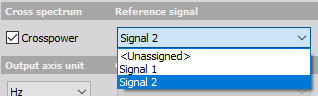### Output quantity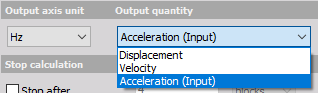Frequency integration/derivation is possible direclty in FFT analyser. If the input quantity is acceleration and we select output quantity as dispacement, the FFT analyser will preform double integration in frequency domain.

The output axis using can be selected from:

• Hz
• rpm - the X axis will have RPM units ([Hz] * 60).
• cpm - the X axis will have cpm units (counts per minute [Hz * 60]).

## Stop calculation

With Stop calculation function you can acquire only a selected number of blocks (or seconds) of data. When the condition is reached, the FFT spectrum will stop updating.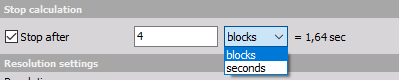## Calculation type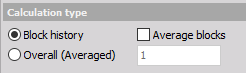• Block history - uses blocks to calculate FFT spectrum.
• Overall (Averaged) - gives only one averaged FFT spectrum at the end of the measurement. It will average all the blocks in the signal and the output will be only one FFT for the whole measurement.

### Averaging type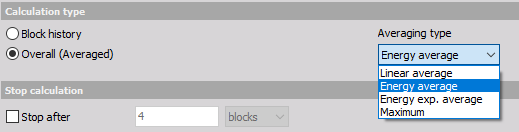When Averaged FFT is selected, there are 4 different averaging types available:

• Linear average - linear average calculates the arithmetic mean of all the values. Linear average gives the same weight to each amplitude value in the average.
• Energy average The energy average is the default average type for stationary measurements. The energy average calculates the averages of the squared values of all values. For this reason, sometimes the term RMS (root mean square) average is used.
• Energy exp. average The energy exponential averages the values and weights the acquisitions taken later in time more heavily than the earlier acquisitions.
• Maximum - maximum value average takes the maximum value at the spectral line. Often this is referred to as peak hold averaging. The maximum value average is useful for measuring the worst case vibration.

## Window function

Window - Rectangular, Hanning, Hamming, Flat Top, Triangle, Blackman, Exponent down, Transient and Backman-Harris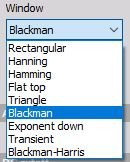## Resolution settings

Resolution - defined in the number of Lines, delta frequency Df [Hz] or with Block duration [s].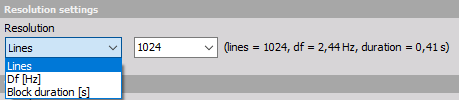## DC cutoff and Overlap

To remove DC or low-frequency components, select from a drop-down list the DC cutoff filter - lower limit.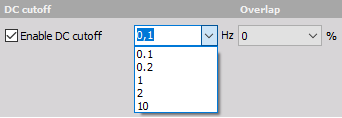Overlap defines the percentage of time signal that has already been calculated and it is used again for calculation (example: 50% overlapping means that the calculation will take half of the old data).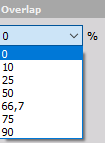## FFT markers

2D graph can display values of the currently selected point with the crosshair cursor. When clicking on such point with the left mouse button, the marker line will be added showing x axis value on the x-axis and showing y-axis vale of a certain point above the marked point. All points can be removed by pressing the right mouse button and select Delete selected marker.

For detailed information about FFT markers see -> 2D Graph.

For additional help visit Dewesoft PRO training  -> Dewesoft Web page -> PRO Training -> FFT markers.

For detailed information about FFT Analysis see -> FFT Analysis.

## Output scalars

The option output scalars will ouput a scalar (a number) that will be calculated in a selected frequency range.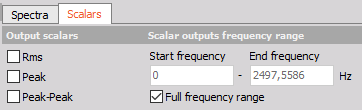• RMS
• Peak
• Peak-Peak

For example, if RMS is selected as the output scalar and whole frequency range is selected, the output channels is Overall RMS value.

## Harmonics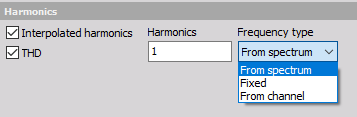THD - Total Harmonic Distortion is a useful technique to analyze any non‑linear behavior of a system.

The frequency type can be from spectrum, fixed frequency or from input channel.

## FFT on asynchronous data

Frequency analysis can be done also on asynchronous input data in the Dewesoft FFT analyzer.

When you select an asynchronous channel as the input, all the synchronous are locked out in the same instance.

You can even select multiple async signals in the same interface, as long as they have the same time base.

There is no resampling done async data when doing frequency analysis.

FFT analyzer recognizes asynchronous data and assumes the samples are equidistant and that the async rate is set properly (the distance between the samples is determined by the rate on channels).

If the data are coming randomly, the signal is not appropriate for analysis.

## FFT on vector data

Frequency analysis can be done also on asynchronous input data in the Dewesoft FFT analyzer.

On vector channels that are input into the FFT analyzer, the user must set the axis correctly.

From the axis definition, the module calculates the frequency.